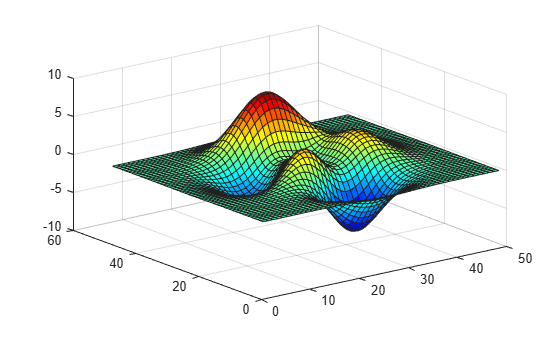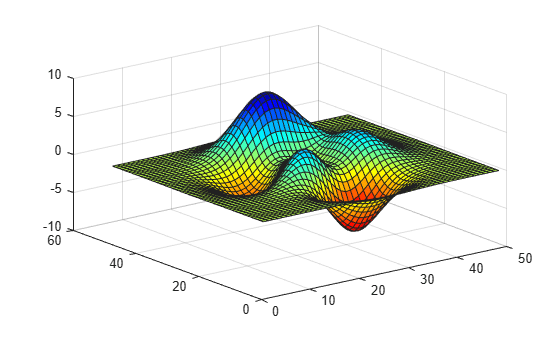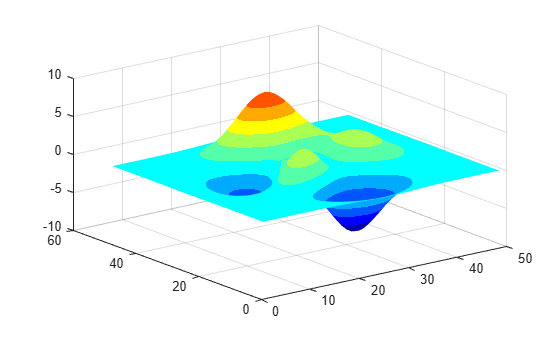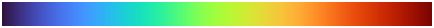# jet

Jet colormap array

## Syntax

``c = jet``
``c = jet(m)``

## Description

example

````c = jet` returns the jet colormap as a three-column array with the same number of rows as the colormap for the current figure. If no figure exists, then the number of rows is equal to the default length of 256. Each row in the array contains the red, green, and blue intensities for a specific color. The intensities are in the range [0,1], and the color scheme looks like this image.For smoother transitions between colors, use the `turbo` colormap. For more information, see Alternative Functionality.```

example

````c = jet(m)` returns the colormap with `m` colors.```

## Examples

collapse all

Plot a surface and assign the jet colormap.

```surf(peaks); colormap('jet');```Get the jet colormap array and reverse the order. Then apply the modified colormap to the surface.

```c = jet; c = flipud(c); colormap(c);```Get a downsampled version of the jet colormap containing only ten colors. Then display the contours of the peaks function by applying the colormap and interpolated shading.

```c = jet(10); surf(peaks); colormap(c); shading interp;```## Input Arguments

collapse all

Number of colors, specified as a scalar integer value. The default value of `m` is equal to the length of the colormap for the current figure. If no figure exists, the default value is 256.

Data Types: `single` | `double`

## Alternative Functionality

### Use the `turbo` Colormap for Smoother Transitions

The `turbo` colormap has a color scheme that is similar to the `jet` colormap, but the transitions between colors are more perceptually uniform.

These colorbars show the difference between the `jet` and `turbo` colormaps.

 `jet``turbo`## Version History

Introduced before R2006a

expand all